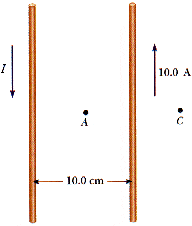# Magnetic Field between two wires

## Homework Statement

Two parallel conductors carry currents in opposite directions, as shown in Figure P19.56. One conductor carries a current of 10.0 A. Point A is the midpoint between the wires, and point C is 5.00 cm to the right of the 10.0 A current. I is adjusted so that the magnetic field at C is zero.Find the value of the magnetic field at A.

## Homework Equations

B = (uI)/(2pi(r))

## The Attempt at a Solution

I actually got the solution. I know that I have to add the field produce by the two currents together. so (uI)/(2pi(.05)) + (uI1)/(2pi(.05)) = B
But can anyone explain why I have to add them together instead of subtracting them? I figured since that current are in opposite direction I would have to subtract them. Thank You